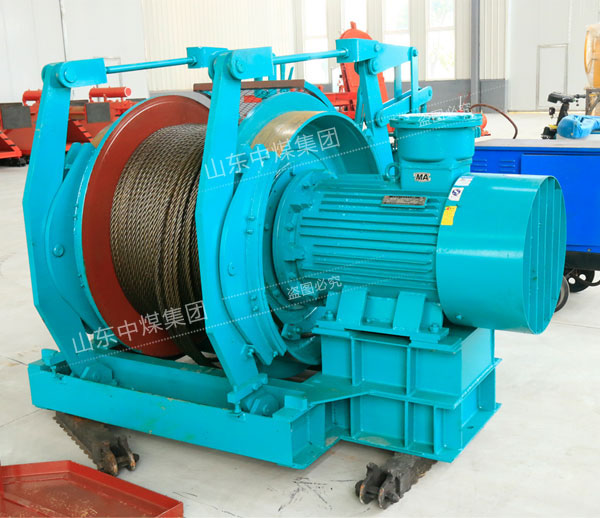# 中煤集团 - 绞车生产制造厂家

http://www.zmjiaoche.com/

# 怎么挑选调度绞车？

调度绞车选型：

绞车的选型依据轨道下山绞车运行斜坡长度、坡度及牵引载荷的重量而定，绞车的牵引力必须大于载荷重量。

牵引载荷：

A =sin6°(Qz+ Q)×9.8×4

=0.1(3000+1500)×9.8×4

=17640N

=17.64KN

查JD-2型绞车牵引力为：20KN>17.64KN,故可选型于JD-2型绞车。

钢丝绳直径为18.5mm。

JD-2型绞车钢丝算：

安全系数： m=ρh /Qj

m ——钢丝绳的安全系数

ρh ——钢丝绳破断力总和

Qj ——钢丝绳静拉力

Qj=g .(Qz+Q)n×(sinθ+f1cosθ)+g.q.L(sinθ+ f2cosθ)

=9.8×(3000+1500)×4×(sin6°+0.02cos6°) +9.8×1.2×200×(sin6°+0.2cos6°

=21833.616N

安全系数m=ρh/Qj=166180/21833.616= 7.6>6.5

式中：

A---斜坡轨道运行时的牵引载荷

Qj ---钢丝绳静拉

Qz---矿车自重：Qz=1500kg

Q----矿车载重：Q=3000kg

n---牵引矿车数辆：n=4

θ--巷道坡度：θ= 6°(sin6°=0.1 cos6°=0.99)

q---钢丝绳每米重量：q=1.2kg/m

L---钢丝绳长度：L=200m

m---钢丝绳的安全系数：

m= 6.5

ρh---钢丝绳破断力：取166.18KN

f1-矿车运动的阻力系数：(f1=0.01-0.02)取0.02

f2-钢丝绳与底板和托辊间的摩擦系数：(f2=0.15-0.2)取0.2。

经过计算，安全系数7.6>6.5符合煤矿安全规程规定，故选用φ=18.5mm的钢丝绳。故选用JD-2型调度绞车进行运输符合要求，你学会了吗？